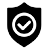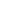#### WhatsApp Quick Chat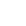#### Get Instant Call Back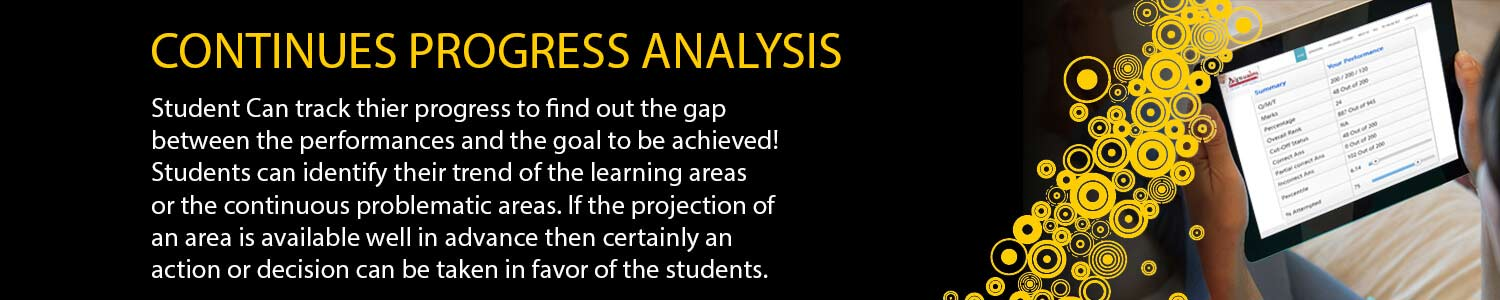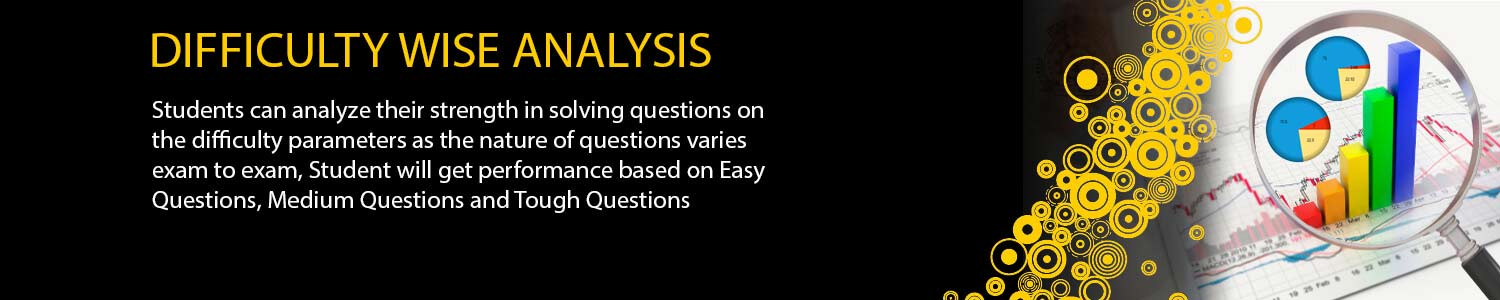## GATE MATH

#### Offers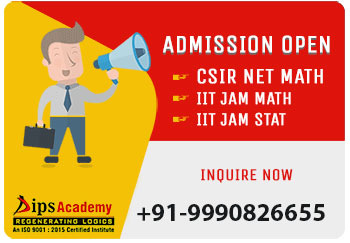##### News & Events# GATE Math Online Test Series

Now a day's most of the exams are being conducted online and students are bound to appear in these exams like GATE and JAM only. We at dips academy are having one of the best online test platforms in India. We use online test application not only to provide the real experience of actual exams but also to give our students the best possible analysis and assessment of their tests.

All the regular students of dips academy are provided their ID and password. Using these credential students not only take online tests, but also get a comprehensive analysis of even those tests which are taken in the classroom on OMR sheets.

Students from all over India can avail our online test facilities. Buying the test package of their requirements, students can not only assess their preparation, but also check their all India rank. Moreover, all the data of classroom tests of the regular students is merged with online test data. So if you are taking online test series from dips academy you can always test your standing with the academy's regular and serious candidates.

##### HIGHLIGHTS

International quality of questions.

The question papers are designed as per the actual importance of the topic in the real scenario.

The questions available on even every minute concept. Practicing such question will provide you the understanding of the concept.

Full length, Topic Wise and concept wise tests available.

Get Answer and solution post test

Similar test screen to actual exam pattern

Flexible to attempt anytime anywhere,just a click away.#### GATE Real Time

(Test Series)#### GATE All India Ranker

(Online Test Series)#### GATE Anytime

(Online Test Series)

### GATE MATHS TEST SERIES SCHEDULE 2023

TEST TYPE Modules DATE Syllabus
MWT-01 Topology + Functional 1-Jan-23 Normed linear spaces, Banach spaces, Hahn-Banach theorem, open mapping and closed graph theorems, principle of uniform boundedness; Inner-product spaces, Hilbert spaces, orthonormal bases, projection theorem, Riesz representation theorem, spectral theorem for compact self-adjoint operators. Basic concepts of topology, bases, subbases, subspace topology, order topology, product topology, quotient topology, metric topology, connectedness, compactness, countability and separation axioms, Urysohn’s Lemma.
MWT-02 LPP 4-Jan-23 Linear programming models, convex sets, extreme points; Basic feasible solution, graphical method, simplex method, two phase methods, revised simplex method ; Infeasible and unbounded linear programming models, alternate optima; Duality theory, weak duality and strong duality; Balanced and unbalanced transportation problems, Initial basic feasible solution of balanced transportation problems (least cost method, north-west corner rule, Vogel’s approximation method); Optimal solution, modified distribution method; Solving assignment problems, Hungarian method.
MWT-03 Real Analysis 07-Jan-23 Metric spaces, connectedness, compactness, completeness; Sequences and series of functions, uniform convergence, Ascoli-Arzela theorem; Weierstrass approximation theorem; contraction mapping principle, Power series; Differentiation of functions of several variables, Inverse and Implicit function theorems; Lebesgue measure on the real line, measurable functions; Lebesgue integral, Fatou’s lemma, monotone convergence theorem, dominated convergence theorem. Functions of two or more variables, continuity, directional derivatives, partial derivatives, total derivative, maxima and minima, saddle point, method of Lagrange’s multipliers
MWT-04 Integra Calculus 10-Jan-23 Functions of two or more variables, continuity, directional derivatives, partial derivatives, total derivative, maxima and minima, saddle point, method of Lagrange’s multipliers; Double and Triple integrals and their applications to area, volume and surface area;
MWT-05 PDE 13-Jan-23 Method of characteristics for first order linear and quasilinear partial differential equations; Second order partial differential equations in two independent variables: classification and canonical forms, method of separation of variables for Laplace equation in Cartesian and polar coordinates, heat and wave equations in one space variable; Wave equation: Cauchy problem and d'Alembert formula, domains of dependence and influence, nonhomogeneous wave equation; Heat equation: Cauchy problem; Laplace and Fourier transform methods.
MWT-06 Mordern Algebra 16-Jan-23 Groups, subgroups, normal subgroups, quotient groups, homomorphisms, automorphisms; cyclic groups, permutation groups, Group action, Sylow’s theorems and their applications;
MWT-07 Vector Calculus 19-Jan-23 Vector Calculus: gradient, divergence and curl, Line integrals and Surface integrals, Green’s theorem, Stokes’ theorem, and Gauss divergence theorem.
MWT-08 Linear Agebra 22-Jan-23 Finite dimensional vector spaces over real or complex fields; Linear transformations and their matrix representations, rank and nullity; systems of linear equations, characteristic polynomial, eigenvalues and eigenvectors, diagonalization, minimal polynomial, Cayley-Hamilton Theorem, Finite dimensional inner product spaces, Gram-Schmidt orthonormalization process, symmetric, skew-symmetric, Hermitian, skew-Hermitian, normal, orthogonal and unitary matrices; diagonalization by a unitary matrix, Jordan canonical form; bilinear and quadratic forms.
MWT-09 Ring Theory 25-Jan-23 Rings, ideals, prime and maximal ideals, quotient rings, unique factorization domains, Principle ideal domains, Euclidean domains, polynomial rings, Eisenstein’s irreducibility criterion; Fields, finite fields, field extensions, algebraic extensions, algebraically closed fields
MWT-10 Complex Analysis 28-Jan-23 Functions of a complex variable: continuity, differentiability, analytic functions, harmonic functions; Complex integration: Cauchy’s integral theorem and formula; Liouville’s theorem, maximum modulus principle, Morera’s theorem; zeros and singularities; Power series, radius of convergence, Taylor’s series and Laurent’s series; Residue theorem and applications for evaluating real integrals; Rouche’s theorem, Argument principle, Schwarz lemma; Conformal mappings, Mobius transformations.
MWT-11 ODE 31-Jan-23 First order ordinary differential equations, existence and uniqueness theorems for initial value problems, linear ordinary differential equations of higher order with constant coefficients; Second order linear ordinary differential equations with variable coefficients; Cauchy-Euler equation, method of Laplace transforms for solving ordinary differential equations, series solutions (power series, Frobenius method); Legendre and Bessel functions and their orthogonal properties; Systems of linear first order ordinary differential equations, Sturm's oscillation and separation theorems, Sturm-Liouville eigenvalue problems, Planar autonomous systems of ordinary differential equations: Stability of stationary points for linear systems with constant coefficients, Linearized stability, Lyapunov functions.
MWT-12 Numerical Analysis 02-Feb-23 Systems of linear equations: Direct methods (Gaussian elimination, LU decomposition, Cholesky factorization), Iterative methods (Gauss-Seidel and Jacobi) and their convergence for diagonally dominant coefficient matrices; Numerical solutions of nonlinear equations: bisection method, secant method, Newton-Raphson method, fixed point iteration; Interpolation: Lagrange and Newton forms of interpolating polynomial, Error in polynomial interpolation of a function; Numerical differentiation and error, Numerical integration: Trapezoidal and Simpson rules, Newton-Cotes integration formulas, composite rules, mathematical errors involved in numerical integration formulae; Numerical solution of initial value problems for ordinary differential equations: Methods of Euler, Runge-Kutta method of order 2
FLT-01 Full Length Test 04-Feb-23 As per Exam Pattern
FLT-02 Full Length Test 06-Feb-23 As per Exam Pattern
FLT-03 Full Length Test 08-Feb-23 As per Exam Pattern
• > Total Questions: 40 (Each MWT) & 55 (Each FLT)

• > Total Marks: 65 (Each MWT) & 85 (Each FLT)

• > Total Duration: 120 Minutes (Each MWT) & 180 Minutes (Each FLT)

## Offers## News & Events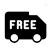##### Free Delivery